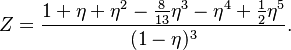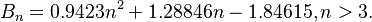2022: SklogWiki celebrates 15 years on-line

Difference between revisions of "Liu hard sphere equation of state"$Z = \frac{ 1 + \eta + \eta^2 - \frac{8}{13}\eta^3 - \eta^4 + \frac{1}{2}\eta^5 }{(1-\eta)^3 }.$$B_n = 0.9423n^2 + 1.28846n - 1.84615, n > 3.$